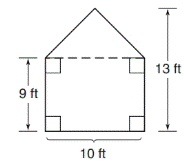Chapter 8.1, Problem 16E### Elementary Geometry for College St...

6th Edition
Daniel C. Alexander + 1 other
ISBN: 9781285195698

#### Solutions

Chapter
Section### Elementary Geometry for College St...

6th Edition
Daniel C. Alexander + 1 other
ISBN: 9781285195698
Textbook Problem
1 views

# In Exercises 9 to 18, find the areas of the figures shown or described.To determine

To find:

The area of given figure.

Explanation

1) Formula:

Finding the area of the figure is followed by identifying the figure and find area with the suitable geometry formulae,

Area of rectangle A=lw unit2

Where, l is the length of rectangle and w is the width of rectangle.

Area of triangle A=12bh unit2

Where, b is the base of triangle and h is the height of triangle.

2) Calculation:

Given,

This is the compound figure of rectangle and triangle.

Area of given figure(A)=Area of rectangle(A1)+Area of a triangle(A2)

Area of rectangle:

A1=lw unit2

Where, l is the length of rectangle 10 ft

w is the width of rectangle 9 ft

A1=10(9)

A1=90 ft2

Area of triangle:

A2=12bh unit2

### Still sussing out bartleby?

Check out a sample textbook solution.

See a sample solution

#### The Solution to Your Study Problems

Bartleby provides explanations to thousands of textbook problems written by our experts, many with advanced degrees!

Get Started

#### Find more solutions based on key concepts